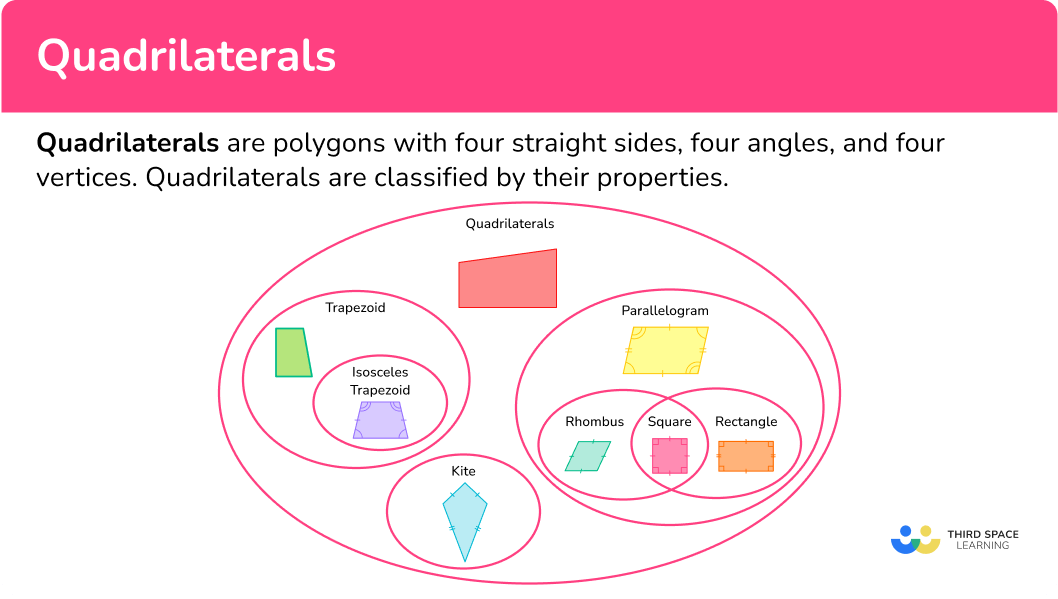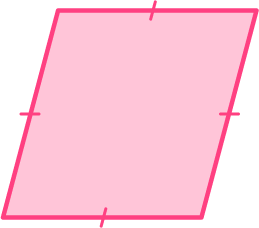Math resources Geometry

Here you will learn about quadrilaterals, including their names, attributes and how to classify them.

Students first learn about quadrilaterals in early elementary school with their work in geometry. They expand upon the types of quadrilaterals as they progress through elementary school.

Quadrilaterals are polygons with four straight sides, four angles, and four vertices.

Here are the special types of quadrilaterals.

NameShapeAttributes
Square

● Four right angles
● Four congruent sides
● Two pairs of opposite parallel sides

Step-by-step guide: Square shape

Rectangle

● Two pairs of opposite congruent sides
● Two pairs of opposite parallel sides
● Four right angles

Step-by-step guide: Rectangle

Parallelogram

● Two pairs of opposite congruent sides
● Two pairs of opposite congruent angles
● Two pairs of opposite parallel sides

Step-by-step guide: Parallelogram

Rhombus

● Four congruent sides
● Two pairs of opposite parallel sides
● Two pairs of opposite congruent angles

Step-by-step guide: Rhombus

Trapezoid

● One pair of opposite parallel sides called bases

Isosceles Trapezoid

● Non-parallel sides are congruent
● Two pairs of congruent angles

Step-by-step guide: Trapezoid

Kite

● Two pairs of adjacent sides that are congruent (next to each other)

To help remember the properties of quadrilaterals and how they relate to one another. You can use a Venn diagram.

Diagonals:

Quadrilaterals have two diagonals. Diagonals are line segments that connect non-consecutive vertices.

• The sum of the interior angles of a quadrilateral total 360 ^{\circ}.

• Quadrilaterals can be convex or concave.

⟶ A convex quadrilateral is a polygon with all interior angles less than 180^{\circ}. All quadrilaterals shown in the table above are convex.

⟶ A concave quadrilateral has at least one angle that is greater than 180^{\circ} (or a reflex angle). The quadrilateral shown below is concave.## Common Core State Standards

How does this relate to 2 nd grade – 5 th grade math?

• Grade 2 – Geometry (2.G.A.1)
Recognize and draw shapes having specified attributes, such as a given number of angles or a given number of equal faces. Identify triangles, quadrilaterals, pentagons, hexagons, and cubes.

• Grade 3 – Geometry (3.G.A.1)
Understand that shapes in different categories (example, rhombuses, rectangles, and others) may share attributes (example, having four sides), and that the shared attributes can define a larger category (example, quadrilaterals). Recognize rhombuses, rectangles, and squares as examples of quadrilaterals, and draw examples of quadrilaterals that do not belong to any of these subcategories.

• Grade 4 – Geometry (4.G.A.2)
Classify two-dimensional figures based on the presence or absence of parallel or perpendicular lines, or the presence or absence of angles of a specified size. Recognize right triangles as a category, and identify right triangles.

• Grade 5 – Geometry (5.G.3)
Understand that attributes belonging to a category of two-dimensional figures also belong to all subcategories of that category. For example, all rectangles have four right angles and squares are rectangles, so all squares have four right angles.

• Grade 5 – Geometry (5.G.4)
Classify two-dimensional figures in a hierarchy based on properties.

In order to name a quadrilateral:

1. Examine the properties of the quadrilateral, including side and angle relationships.

### Example 1: parallelogram

The quadrilateral has two pairs of parallel sides. Identify the type of quadrilateral that it is.

1. Examine the properties of the quadrilateral, including side and angle relationships.

The quadrilateral has two pairs of parallel sides. It also appears that the quadrilateral has two pairs of opposite congruent sides and two pairs of opposite congruent angles.

### Example 2: rectangle

What is the best classification for the quadrilateral?

The sides of the quadrilateral are not all equal. The quadrilateral has two pairs of opposite congruent sides, two pairs of opposite parallel sides, and four equal angles – right angles.

The best classification for the quadrilateral is a rectangle. Rectangles are special types of parallelograms.

Select the regular quadrilateral and name it.

Choice C is the regular quadrilateral because all of the sides are congruent and all angles are congruent. The regular quadrilateral is a square.

### Example 4: rhombus

Kelly wants to classify the quadrilateral. What is the best classification?

The quadrilateral has two pairs of parallel sides as well as all four congruent sides. All four angles are not congruent.

The quadrilateral is a rhombus. Rhombuses are special types of parallelograms.

### Example 5: trapezoid

The quadrilateral has one pair of opposite sides that are parallel.

Describe how the quadrilaterals are the same and how they are different. Name them.

Quadrilateral A has two pairs of opposite parallel sides and two pairs of opposite congruent sides. Quadrilateral A also has two pairs of opposite congruent angles.

Quadrilateral B has one pair of opposite parallel sides and two pairs of congruent angles. Quadrilateral B also has one pair of opposite congruent sides.

The quadrilaterals are the same because they have a pair of opposite parallel sides, a pair of opposite congruent sides, and two pairs of congruent angles.

They are different because Quadrilateral A has two pairs of opposite parallel sides and Quadrilateral B only has one pair.

Quadrilateral A has two pairs of opposite congruent sides, and quadrilateral B only has one. Also, Quadrilateral A has two pairs of opposite angles that are congruent, whereas Quadrilateral B has adjacent angles (next to each other) that are congruent.

Quadrilateral B is an isosceles trapezoid.

• Have students create a hierarchy of quadrilaterals on their own so that they can investigate the relationships between the sides of a quadrilateral and the angles of a quadrilateral.

• Instead of worksheets, have students engage with geometric digital platforms such as desmos to investigate the properties of quadrilaterals.

• Incorporate project based learning activities which include ways for students to see how the concepts are applied in a real world setting.

### Easy mistakes to make

• Assuming all quadrilaterals with four \bf{90}^{\circ} angles are squares
Rectangles and squares are quadrilaterals with four right angles. If the four sides are equal, then it is a square. If the four sides are not equal, then it is a rectangle.

• Mistaking a trapezoid for a parallelogram
Trapezoids are not classified as parallelograms because they do not hold the properties of parallelograms. Trapezoids have only one pair of opposite parallel sides. Parallelograms have two pairs of opposite parallel sides.

• Mistaking a rhombus for a square
A rhombus has four equal sides, but its angles are not right angles like in a square.rectangletrapezoidsquarerhombusThe quadrilateral has four congruent sides and the four angles are not congruent. So, the quadrilateral is a rhombus.

2. Which quadrilateral has two pairs of opposite parallel sides and four right angles, but does NOT have all sides of equal length?

RhombusRectangleParallelogramSquareQuadrilaterals with two pairs of opposite parallel sides with four right angles have to be a special parallelogram, which is either a rectangle or a rhombus. Since the quadrilateral does not have four congruent sides, it must be a rectangle.

3. Which quadrilateral is NOT a parallelogram?The only quadrilateral that cannot be a parallelogram from the choices is the trapezoid.A trapezoid has only one pair of opposite parallel sides whereas parallelograms have two pairs of opposite parallel sides.

4. Which statement is true?

A square is always a rhombus.A rhombus is always a square.A trapezoid is always a parallelogram.A parallelogram is always a trapezoid.A square is always considered a rhombus because, in order for a quadrilateral to be a rhombus, it has to have 4 congruent sides. Squares have four congruent sides.

However, a rhombus is not always a square because in order for a quadrilateral to be a square it has to have four congruent sides and four right angles. Rhombuses do not have four right angles.

5. Which quadrilateral could have side lengths 10 \, cm, 6 \, cm, 10 \, cm, 6 \, cm?

RhombusParallelogramTrapezoidSquareParallelograms have two pairs of opposite parallel sides and two pairs of opposite congruent sides. So it is the only quadrilateral choice whose side lengths can be 10 \, cm, 6 \, cm, 10 \, cm and 6 \, cm.

6. The quadrilateral below can be classified as which of the following?a parallelogram and a rectanglea rectangle and a rhombusa rectangle and a squarea parallelogram and a rhombusA rhombus has two pairs of opposite parallel sides and two pairs of opposite congruent angles which makes it a parallelogram. It also has four congruent sides making it a special type of parallelogram.

What is another name for a quadrilateral?

A quadrilateral is also a four-sided polygon or tetragon.

What is a trapezium?

A trapezium is another name for a trapezoid.

Do the diagonals of quadrilaterals have special properties?

You will study in detail the diagonals of quadrilaterals in middle and high school. However, they do hold special properties. The diagonals of parallelograms bisect each other.

In the special cases of parallelograms, such as rectangles, the diagonals are equal in length, in rhombuses, the diagonals are perpendicular, and in squares, the diagonals are perpendicular bisectors. Kites also have perpendicular diagonals.